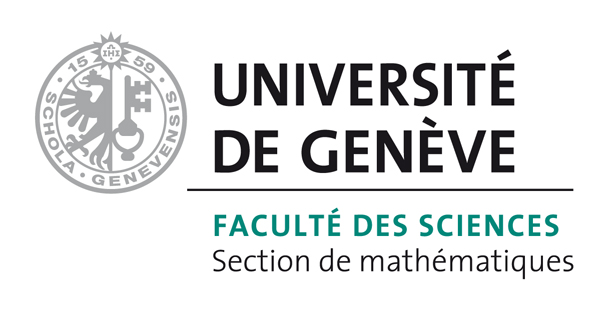# Bart Vandereycken## Geometric methods on low-rank matrix and tensor manifolds

by ,

#### Abstract:

In this chapter we present numerical methods for low-rank matrix and tensor problems that explicitly make use of the geometry of rank constrained matrix and tensor spaces. We focus on two types of problems: The first are optimization problems, like matrix and tensor completion, solving linear systems and eigenvalue problems. Such problems can be solved by numerical optimization for manifolds, called Riemannian optimization methods. We will explain the basic elements of differential geometry in order to apply such methods efficiently to rank constrained matrix and tensor spaces. The second type of problem is ordinary differential equations, defined on matrix and tensor spaces. We show how their solution can be approximated by the dynamical low-rank principle, and discuss several numerical integrators that rely in an essential way on geometric properties that are characteristic to sets of low rank matrices and tensors.

#### Reference:

A. Uschmajew, B. Vandereycken, "Geometric methods on low-rank matrix and tensor manifolds", Chapter in Variational methods for nonlinear geometric data and applications (P. Grohs, M. Holler, A. Weinmann, eds.), Springer, 2020.

#### Bibtex Entry:

@incollection{Uschmajew_V:2020_b,
Abstract = {In this chapter we present numerical methods for low-rank matrix and tensor problems that explicitly make use of the geometry of rank constrained matrix and tensor spaces. We focus on two types of problems: The first are optimization problems, like matrix and tensor completion, solving linear systems and eigenvalue problems. Such problems can be solved by numerical optimization for manifolds, called Riemannian optimization methods. We will explain the basic elements of differential geometry in order to apply such methods efficiently to rank constrained matrix and tensor spaces. The second type of problem is ordinary differential equations, defined on matrix and tensor spaces. We show how their solution can be approximated by the dynamical low-rank principle, and discuss several numerical integrators that rely in an essential way on geometric properties that are characteristic to sets of low rank matrices and tensors.},
Author = {Uschmajew, A. and Vandereycken, B.},
Title = {Geometric methods on low-rank matrix and tensor manifolds},
Editor = {Grohs, P. and Holler, M. and Weinmann, A.},
Publisher = {Springer},
Booktitle = {Variational methods for nonlinear geometric data and applications},
Year = {2020},
Doi = {10.1007/978-3-030-31351-7_9},
Pdf = {http://www.unige.ch/math/vandereycken/papers/published_Uschmajew_V_2020_b.pdf}}